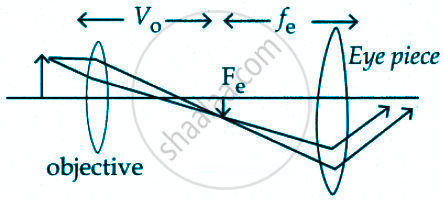Tamil Nadu Board of Secondary EducationHSC Science Class 12th

# A compound microscope has a magnifying power of 100 when the image is formed at infinity. The objective has a focal length of 0.5 cm and the tube length is 6.5 cm. - Physics

Numerical

A compound microscope has a magnifying power of 100 when the image is formed at infinity. The objective has a focal length of 0.5 cm and the tube length is 6.5 cm. What is the focal length of the eyepiece.

#### Solution

"v"_circ + "f"_"e" = 6.5 cm   ....(1)

m = "v"_circ/"u"_circ xx "D"/"f"_"e" [taking D = 25 cm]

=> "m" = - [1 - "v"_circ/"f"_circ] "D"/"f"_"e"

100 = - [1 - "v"_circ/0.5] 25/"f"_"e"

100 "f"_"e" = - (1 - 2"v"_circ) xx 25

2"v"_circ - 4 "f"_"e" = 1   .....(2)

Solving equation (1) and (2) we can get,

v0 = 4.5 cm and fe = 2 cmConcept: Optical Instruments
Is there an error in this question or solution?

Share